# ACEt v1.8.2

## Installation

The R package can be installed from CRAN

install.packages("ACEt")

The installation requires Rcpp-0.11.1 and has been tested on R-4.1.3. The installation of the ACEt package also requires installing the BH and RcppArmadillo packages.

install.packages("devtools")
library(devtools)
install_github("lhe17/ACEt")

## Application to an example dataset

We illustrate how to utilize the ACEt R package with an example dataset that can be loaded with the following codes. More detail about the method is given in He et al. (2016) and He et al. (2017).

library(ACEt)
data(data_ace)

The example dataset contains two matrices mz and dz for MZ and DZ twins, respectively. Each matrix includes 2500 twin pairs, of which the first two columns are the quantitative phenotype of the twin pair and the third column (T_m or T_d) is age.

attributes(data_ace)
#> $names #>  "mz" "dz" head(data_ace$mz)
#>                            T_m
#> [1,]  2.5638027  4.7355457   1
#> [2,] -3.1959902 -3.0133873   1
#> [3,]  1.3924694  2.8656795   1
#> [4,]  2.4519483  1.9145994   1
#> [5,] -0.4186678  1.3470608   1
#> [6,] -1.2805044 -0.5234272   1
head(data_ace$dz) #> T_d #> [1,] 0.1402850 -0.3430456 1 #> [2,] 0.8588600 -0.7381698 1 #> [3,] 0.4025476 0.2794685 1 #> [4,] -0.4564107 0.2008932 1 #> [5,] -0.2458682 -3.0600677 1 #> [6,] 0.1459282 0.4588418 1 The age is distributed uniformly from 1 to 50 in both twin datasets and the phenotypes are normally distributed with a mean equal to zero. As discussed in He et al. (2017), before used as an input for this package, the phenotype should be centered, for example, by using residuals from a linear regression model lm() in which covariates for the mean function can be included. Fitting an ACE(t) model can be done by calling the AtCtEt function, in which users can specify a function (null, constant or splines) for each component independently through the mod argument. # fitting the ACE(t) model re <- AtCtEt(data_ace$mz, data_ace$dz, mod = c('d','d','c'), knot_a = 6, knot_c = 4) summary(re) #> Length Class Mode #> n_beta_a 1 -none- numeric #> n_beta_c 1 -none- numeric #> n_beta_e 1 -none- numeric #> beta_a 7 -none- numeric #> beta_c 5 -none- numeric #> beta_e 1 -none- numeric #> hessian_ap 169 -none- numeric #> hessian 169 -none- numeric #> con 1 -none- numeric #> lik 1 -none- numeric #> knots_a 10 -none- numeric #> knots_c 8 -none- numeric #> knots_e 2 -none- numeric #> min_t 1 -none- numeric #> max_t 1 -none- numeric #> boot 0 -none- NULL In the above script, an ACE(t) model is fitted for the example dataset. The first two arguments specify the matrices of the phenotypes for MZ and DZ twins, respectively. The argument mod = c('d','d','c') specifies that we allow the variances of the A and C components to change dynamically and assume the variance of the E component to be a constant over age. The mod argument is a vector of three elements corresponding to the A, C and E components that can be 'd', 'c' or 'n', in which 'n' represents the exclusion of a component. For example, mod = c('d','n','c') indicates that we fit an AE model with a dynamic A component and a constant E component. It should be noted that the E component cannot be eliminated. We can also give the number of knots for each component, which is ignored if we choose 'c' or 'n' for that component. The number of randomly generated initial values for the estimation algorithm can be specified using the robust argument. Multiple initial values can be attempted to minimize the risk of missing the global maximum. The AtCtEt function returns both analytical and approximated Hessian matrices (shown below), which are close to each other in general and can be used to compute pointwise CIs. Note that the analytical Hessian is always positive definite, but the approximated one is not necessarily positive definite. The returned value lik is the negative log-likelihood that can be used for LRT for the comparison of twin models. # part of the analytical Hessian matrix re$hessian[1:8,1:8]
#>           [,1]      [,2]        [,3]         [,4]       [,5]       [,6]
#> [1,] 3.3122436  8.572181   0.9774334   0.00000000   0.000000   0.000000
#> [2,] 8.5721808 64.560338  43.2299744   2.22206265   0.000000   0.000000
#> [3,] 0.9774334 43.229974 145.2021892  67.94632724   2.836022   0.000000
#> [4,] 0.0000000  2.222063  67.9463272 182.31265682  68.569776   2.334633
#> [5,] 0.0000000  0.000000   2.8360218  68.56977584 155.634291  54.989838
#> [6,] 0.0000000  0.000000   0.0000000   2.33463321  54.989838 113.226897
#> [7,] 0.0000000  0.000000   0.0000000   0.00000000   1.840752  22.878770
#> [8,] 4.9109578 13.518421   2.7632296   0.02669836   0.000000   0.000000
#>           [,7]        [,8]
#> [1,]  0.000000  4.91095781
#> [2,]  0.000000 13.51842124
#> [3,]  0.000000  2.76322956
#> [4,]  0.000000  0.02669836
#> [5,]  1.840752  0.00000000
#> [6,] 22.878770  0.00000000
#> [7,] 11.077766  0.00000000
#> [8,]  0.000000 11.02095804
# part the Hessian matrix approximated by the L-BFGS algorithm
re$hessian_ap[1:8,1:8] #> [,1] [,2] [,3] [,4] [,5] [,6] #> [1,] 3.3066880 8.583804 0.9771341 0.00000000 0.000000 0.000000 #> [2,] 8.5838039 64.611733 43.1402778 2.22883073 0.000000 0.000000 #> [3,] 0.9771341 43.140278 144.8342062 67.91347006 2.836111 0.000000 #> [4,] 0.0000000 2.228831 67.9134701 181.14525247 67.405340 2.312405 #> [5,] 0.0000000 0.000000 2.8361108 67.40534018 155.154944 55.376537 #> [6,] 0.0000000 0.000000 0.0000000 2.31240520 55.376537 114.366468 #> [7,] 0.0000000 0.000000 0.0000000 0.00000000 1.856622 23.555961 #> [8,] 5.0462737 13.315584 2.7042919 0.02561431 0.000000 0.000000 #> [,7] [,8] #> [1,] 0.000000 5.04627369 #> [2,] 0.000000 13.31558433 #> [3,] 0.000000 2.70429192 #> [4,] 0.000000 0.02561431 #> [5,] 1.856622 0.00000000 #> [6,] 23.555961 0.00000000 #> [7,] 11.486909 0.00000000 #> [8,] 0.000000 11.08078500 The AtCtEt function returns the minus log-likelihood evaluated at the estimates that is needed to make inference based on LRT. For example, the following program tests whether the A or C component has a constant variance with respect to age, we fit the null models and calculate the p-values based on $$\chi^2$$ distributions. It can be seen that the LRT has no sufficient statistical power to reject the constancy of the C component with this sample size (p1>0.05). In addition, we test whether the C component can be ignored by comparing re_cc and re_cn and compute the p-value (p3) based on a mixture of $$\chi^2$$ distributions. re_cc <- AtCtEt(data_ace$mz, data_ace$dz, mod = c('d','c','c'), knot_a = 6, knot_c = 4) p1 <- pchisq(2*(re_cc$lik-re$lik), 4, lower.tail=FALSE) p1 #>  0.2079343 re_ac <- AtCtEt(data_ace$mz, data_ace$dz, mod = c('c','d','c'), knot_a = 6, knot_c = 4) p2 <- pchisq(2*(re_ac$lik-re$lik), 6, lower.tail=FALSE) p2 #>  8.937498e-12 re_cn <- AtCtEt(data_ace$mz, data_ace$dz, mod = c('d','n','c'), knot_a = 6, knot_c = 4) p3 <- 0.5*pchisq(2*(re_cn$lik-re_cc$lik), 1, lower.tail=FALSE) p3 #>  2.155026e-08 After fitting the ACE(t) model, we can plot the estimated variance curves by calling the plot_acet function. plot_acet(re, ylab='Var', xlab='Age (1-50)')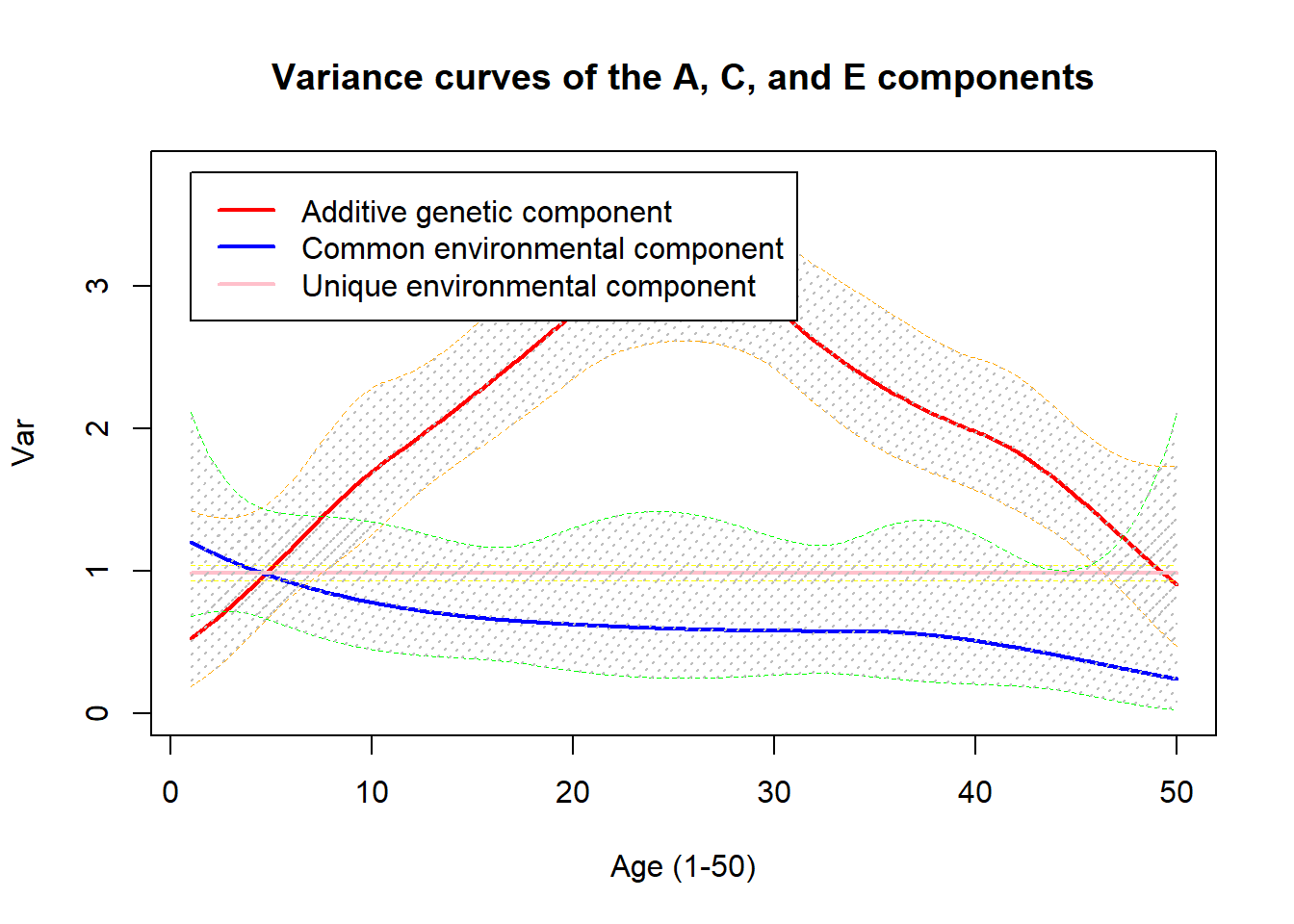By default, the 95% pointwise CIs are estimated using the delta method. Alternatively, we can choose the bootstrap method by setting boot=TRUE and giving the number of bootstrap resampling, the default value of which is 100. ## fitting an ACE(t) model with the CIs esitmated by the bootstrap method re_b <- AtCtEt(data_ace$mz, data_ace$dz, mod = c('d','d','c'), knot_a = 6, knot_c = 4, boot = TRUE, num_b = 60) plot_acet(re_b, boot = TRUE)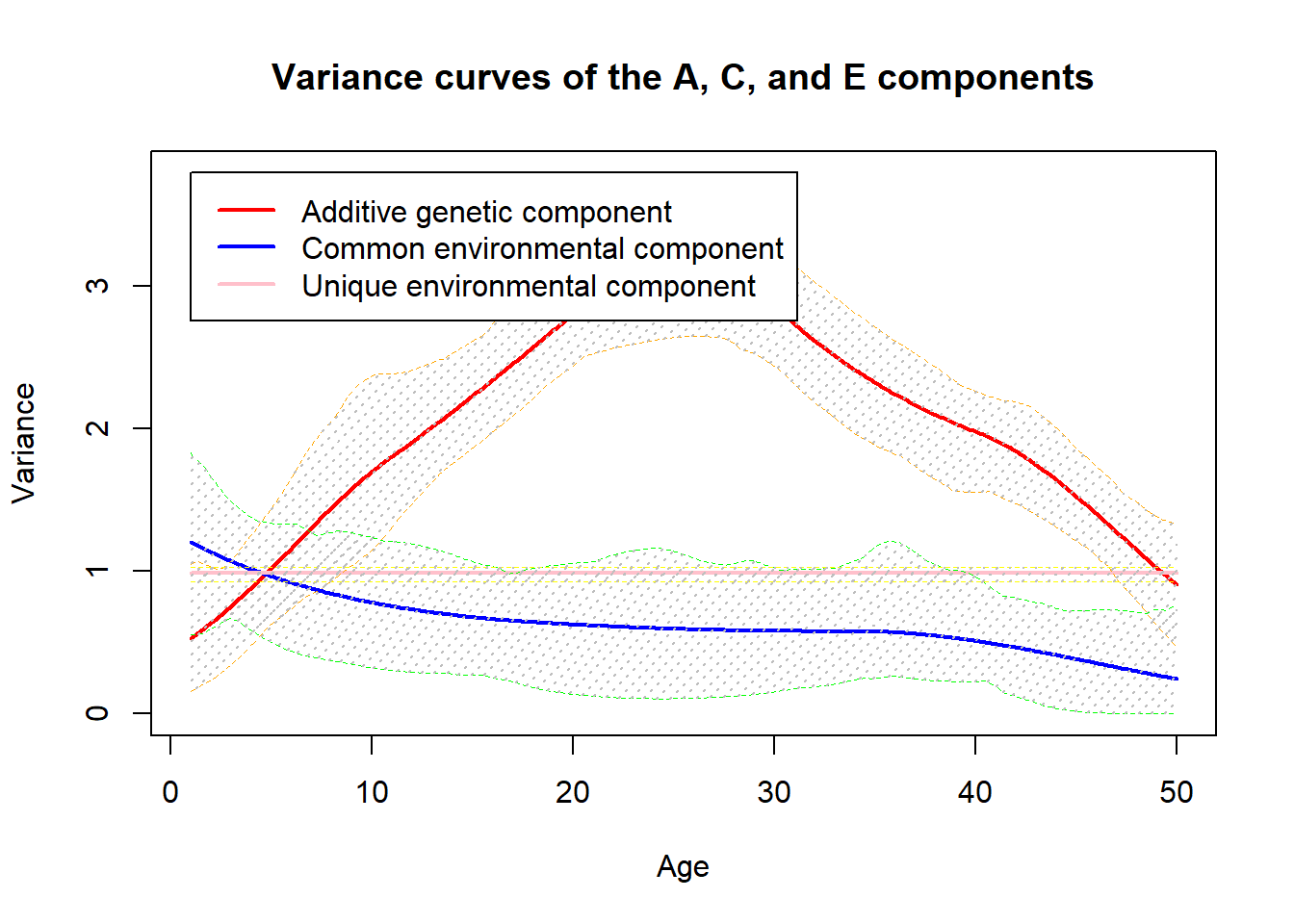Next, we plot the age-specific heritability by setting the argument heri=TRUE in the plot_acet function. And similarly we can choose either the delta method or the bootstrap method to generate the CIs. ## plot dynamic heritability with the CIs using the delta method plot_acet(re_b, heri=TRUE, boot = FALSE)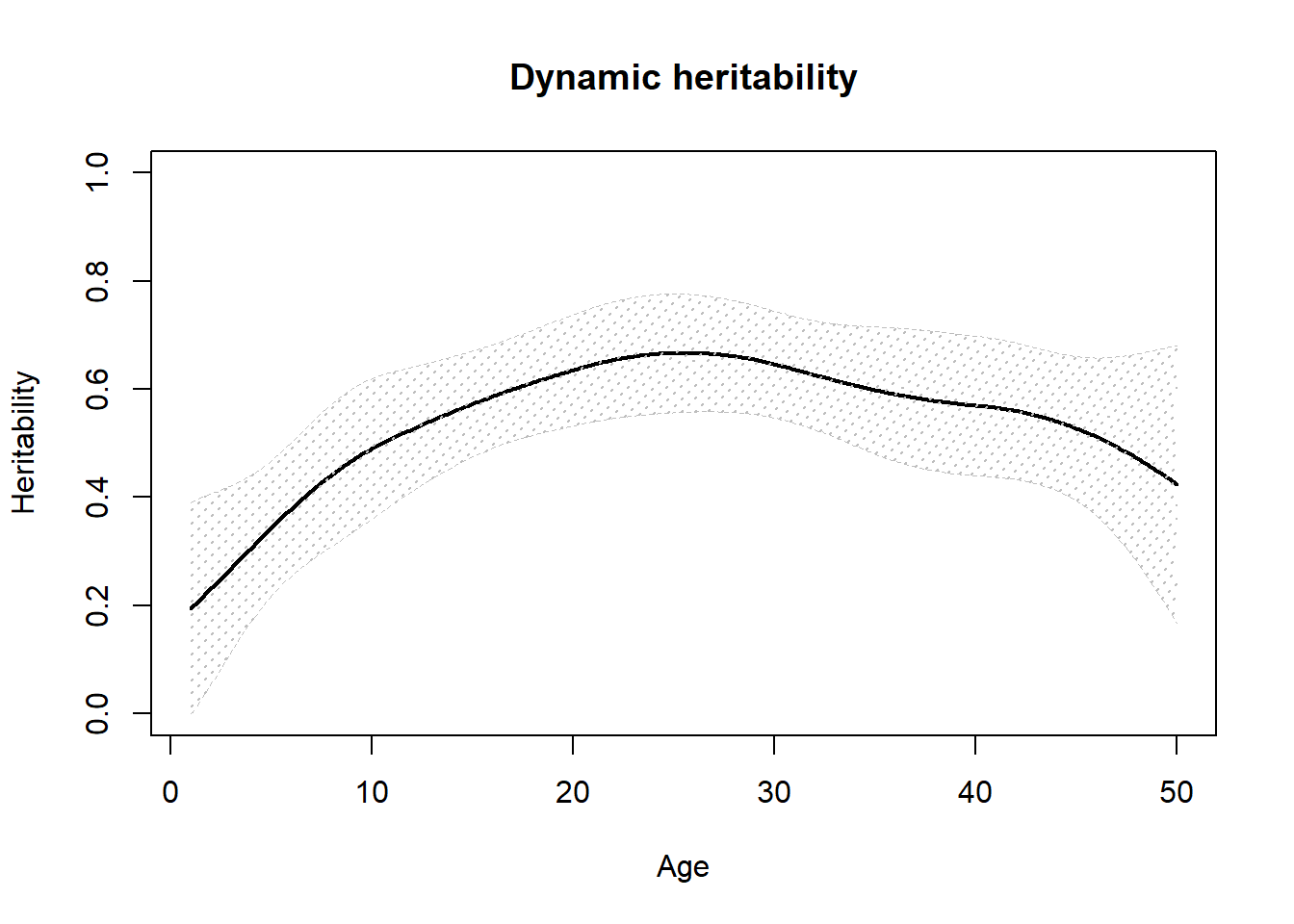## plot dynamic heritability with the CIs using the bootstrap method plot_acet(re_b, heri=TRUE, boot = TRUE)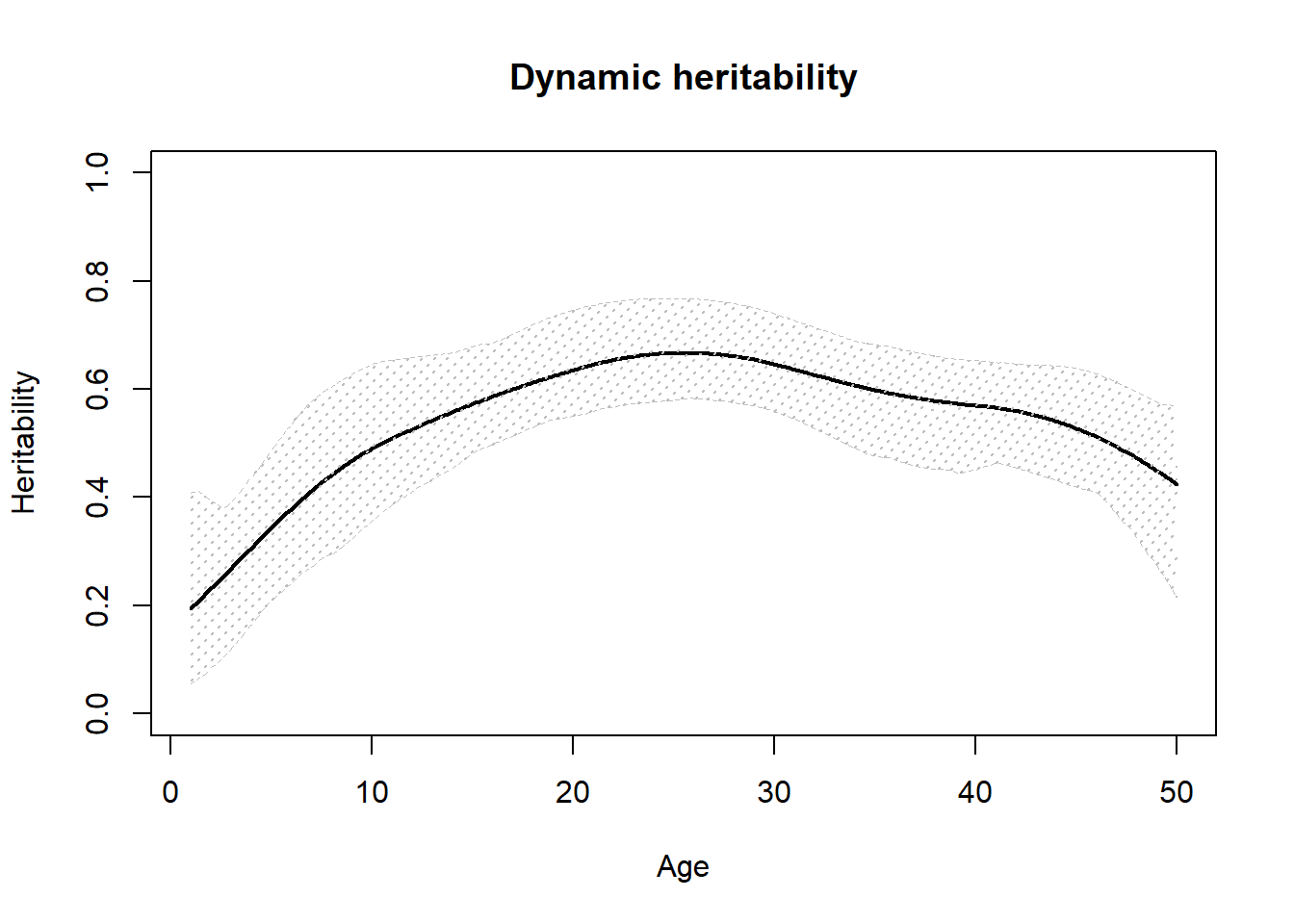The ACE(t)-p model is implemented in the AtCtEtp function, in which users can choose exponential of penalized splines, a linear function or a constant to model a certain component by setting the mod argument. Compared to the ACE(t) model, it is not an essential problem to provide an excessive number of knots (the default value of interior knots is 8) when using the ACE(t)-p model as it is more important to ensure adequate knots for curves with more fluctuation than to avoid overfitting. Below, we fit the example dataset using the AtCtEtp function in which the A and C components are modelled by B-splines of 8 interior knots and the E component by a log-linear function. Similar to the AtCtEt function, we can use the robust argument to specify the number of randomly generated initial values, which can reduce the program’s possibility of being stuck on a local maximum in the EM algorithm. ## fitting an ACE(t)-p model re <- AtCtEtp(data_ace$mz, data_ace\$dz, knot_a = 8, knot_c = 8, mod=c('d','d','l'))
summary(re)
#>         Length Class  Mode
#> D_a       81   -none- numeric
#> D_c       81   -none- numeric
#> D_e        4   -none- numeric
#> pheno_m 5000   -none- numeric
#> pheno_d 5000   -none- numeric
#> T_m     5000   -none- numeric
#> T_d     5000   -none- numeric
#> knot_a    12   -none- numeric
#> knot_c    12   -none- numeric
#> knot_e     2   -none- numeric
#> beta_a     9   -none- numeric
#> beta_c     9   -none- numeric
#> beta_e     2   -none- numeric
#> con        1   -none- numeric
#> lik        1   -none- numeric
#> iter       5   -none- numeric
#> var_b_a    1   -none- numeric
#> var_b_c    1   -none- numeric
#> var_b_e    1   -none- numeric
#> mod        3   -none- character
#> hessian    9   -none- numeric

The AtCtEtp function finds MLE of the variance $$\sigma^{2}_{\beta^{A,C,E}}$$ using the integrated likelihood and also provides estimates of the spline coefficients, i.e. $$\beta^{A,C,E}$$, which are based on maximum a posteriori (MAP) estimation. For a variance component of log-linearity (the E component in this example), $$\beta$$ is a vector of two elements that $$exp(\beta)$$ are the variances of this component at the minimum and maximum age in the dataset. To obtain the empirical Bayes estimates of $$\beta^{A,C,E}$$ and the covariance matrix using the MCMC method, we then call the acetp_mcmc function by plugging the result from the AtCtEtp function. We can also specify the numbers of the MCMC iterations and burn-in.

re_mcmc <- acetp_mcmc(re, iter_num = 5000, burnin = 500)
summary(re_mcmc)
#>           Length Class  Mode
#> beta_a_mc   9    -none- numeric
#> beta_c_mc   9    -none- numeric
#> beta_e_mc   2    -none- numeric
#> cov_mc    400    -none- numeric
#> knots_a    12    -none- numeric
#> knots_c    12    -none- numeric
#> knots_e     2    -none- numeric
#> min_t       1    -none- numeric
#> max_t       1    -none- numeric

Given the esimates together with their covariance matrix, we can plot the variance curves or dynamic heritability by calling the plot_acet function. The boot option is ignored for the ACE(t)-p model.

plot_acet(re_mcmc)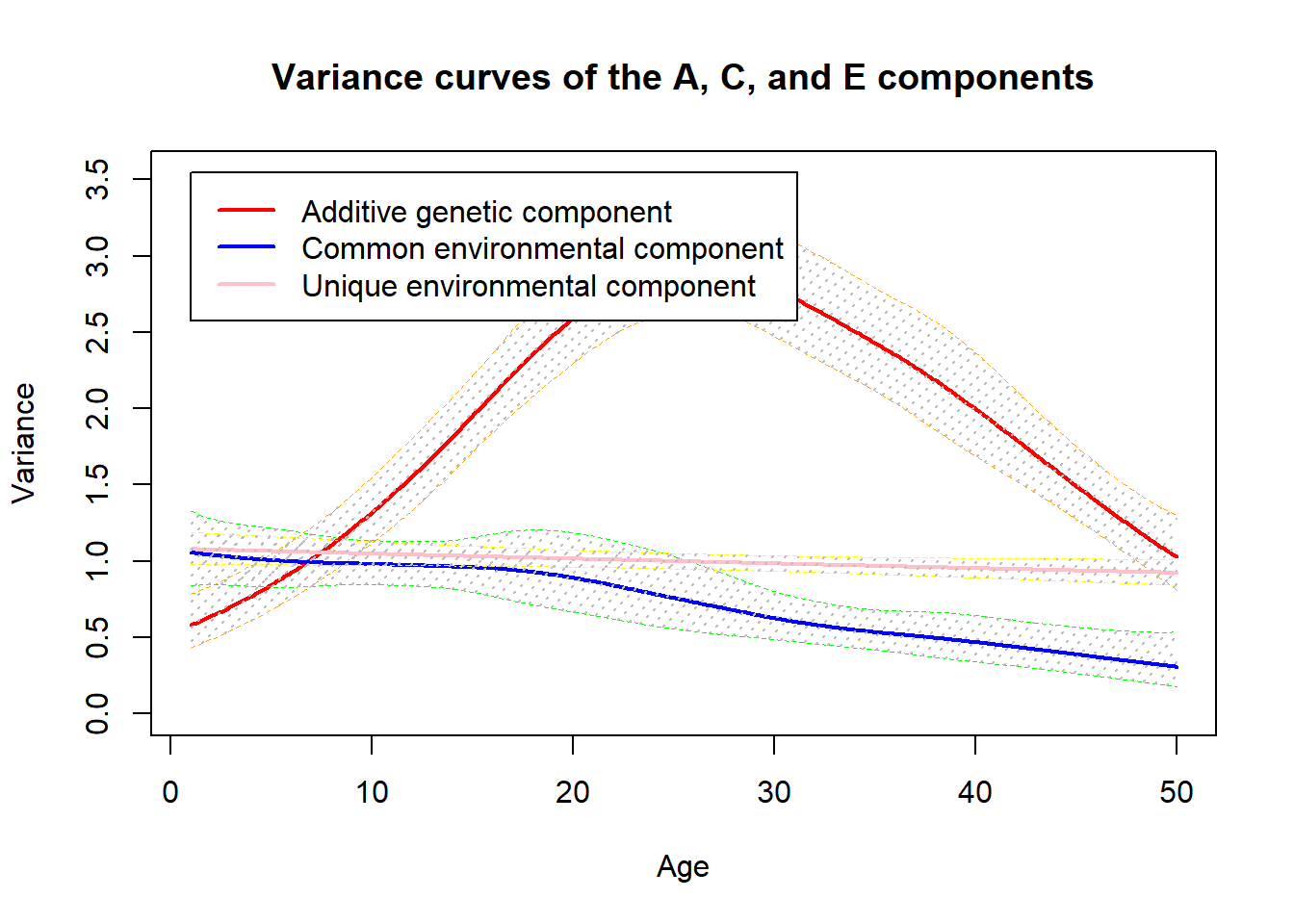plot_acet(re_mcmc, heri=TRUE)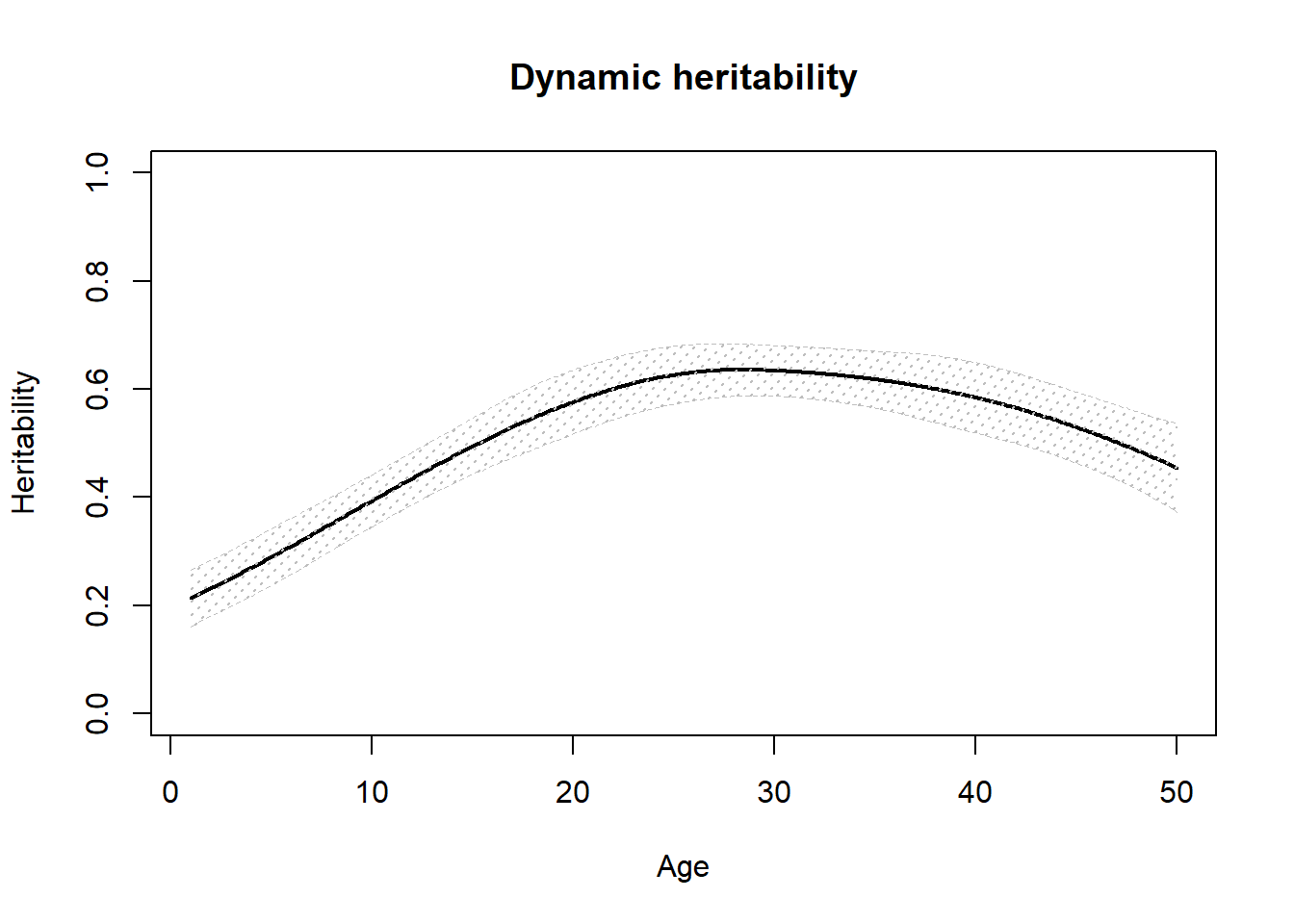Assigning too many knots in the ACE(t)-p model is much less harmful than that in the ACE(t) model. Comparing the following two plots from the application of the two models with 10 knots for each component to the example data set, it suggests that the ACE(t) model has an overfitting problem but the ACE(t)-p model works properly.